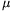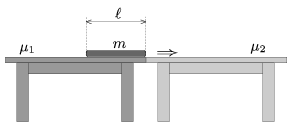Mathematical and Physical Journal
for High Schools
Issued by the MATFUND Foundation
 Already signed up? New to KöMaL?

#Problem P. 4554. (September 2013)

P. 4554. There are two tables next to each other. How much work is done on that flat parcel, which is pulled from one of the tables to the other? The coefficients of dynamic friction between the parcel and the two tabletops are different.

Data: m=18 kg;=0.8 m;1=0.1;2=0.4. ( The weight of the parcel is distributed evenly along the surfaces of the tables that are in contact with the parcel.)(4 pont)

Deadline expired on October 10, 2013.

Sorry, the solution is available only in Hungarian. Google translation

Megoldásvázlat. $\displaystyle W=mg\ell\frac{\mu_1+\mu_2}{2}=35{,}3$ J.

### Statistics:

 219 students sent a solution. 4 points: 178 students. 3 points: 20 students. 2 points: 13 students. 1 point: 2 students. 0 point: 4 students. Unfair, not evaluated: 2 solutionss.

Problems in Physics of KöMaL, September 2013